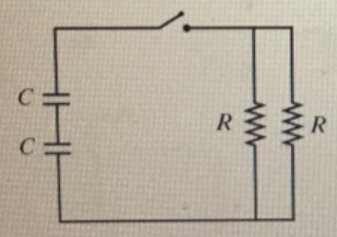# Problem: Suppose that R = 5 kΩ and C = 5μFWhat is the time constant for the discharge of the capacitors in? Express your answer to two significant figures and include approximate units.

###### FREE Expert Solution

Time constant for a RC circuit:

$\overline{){\mathbf{T}}{\mathbf{=}}{\mathbit{R}}{\mathbit{C}}}$

Equivalent resistance for resistors in parallel:

$\overline{)\frac{\mathbf{1}}{{\mathbf{R}}_{\mathbf{e}\mathbf{q}}}{\mathbf{=}}\frac{\mathbf{1}}{{\mathbf{R}}_{\mathbf{1}}}{\mathbf{+}}\frac{\mathbf{1}}{{\mathbf{R}}_{\mathbf{2}}}}$

Equivalent capacitance for capacitors in series:

$\overline{)\frac{\mathbf{1}}{{\mathbf{C}}_{\mathbf{eq}}}{\mathbf{=}}\frac{\mathbf{1}}{{\mathbf{C}}_{\mathbf{1}}}{\mathbf{+}}\frac{\mathbf{1}}{{\mathbf{C}}_{\mathbf{2}}}}$

Req = (1/R1 + 1/R2)-1 = (1/5 + 1/5)-1 = 2.5 kΩ(1000Ω/1kΩ) = 2500 Ω

99% (112 ratings)###### Problem Details

Suppose that R = 5 kΩ and C = 5μF

What is the time constant for the discharge of the capacitors in? Express your answer to two significant figures and include approximate units.Frequently Asked Questions

What scientific concept do you need to know in order to solve this problem?

Our tutors have indicated that to solve this problem you will need to apply the !! Resistor-Capacitor Circuits concept. If you need more !! Resistor-Capacitor Circuits practice, you can also practice !! Resistor-Capacitor Circuits practice problems.

What professor is this problem relevant for?

Based on our data, we think this problem is relevant for Professor Velissaris' class at UCF.